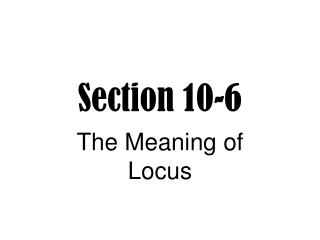# Section 10-6 - PowerPoint PPT PresentationDownload PresentationSection 10-6

Download Presentation## Section 10-6

- - - - - - - - - - - - - - - - - - - - - - - - - - - E N D - - - - - - - - - - - - - - - - - - - - - - - - - - -
##### Presentation Transcript

1. Section 10-6 The Meaning of Locus

2. Locus A figure that is the set of all points, and only those points, that satisfy one or more conditions

3. 1 cm 1 cm 1 cm 1 cm 1 cm k Given line k, find the locus of points that are 1 cm from k in a plane. The locus is a pair of lines parallel to, and 1 cm from, k.

4. 1 cm k Given line k, find the locus of points that are 1 cm from k in space. The locus is a cylindrical surface with axis k and a 1 cm radius.

5. Find the locus of points in a plane 2 cm from circle O with radius 5cm. 2 2 5cm The locus of points is two concentric circles with center O, one with radius 3cm and one with radius 7cm.

6. Find the locus of points in space 2 cm from sphere O with radius 5cm. 2 2 5cm The locus of points is two concentric spheres with center O, one with radius 3cm and one with radius 7cm.

7. Draw and describe the locus of points in a plane that are equidistant from the endpoints of segment AB.

8. Draw and describe the locus of points in space that are equidistant from the endpoints of segment AB.

9. Draw and describe the locus of points in a plane that are equidistant from the sides of angle ABC.

10. Draw and describe the locus of points in a plane that are length AB from line a.

11. Draw and describe the locus of points in space that are length AB from line a.

12. Draw and describe the locus of points in a plane that are midpoints of the radii of circle O with radius 6.

13. Draw and describe the locus of points in space that are midpoints of the radii of sphere O with radius 6.

14. Draw and describe the locus of points in a plane that are equidistant from the endpoints of diameter AB of circle O.

15. Draw and describe the locus of points on or in square WXYZ that are equidistant from all four sides.